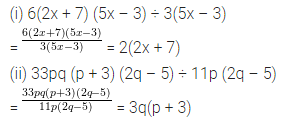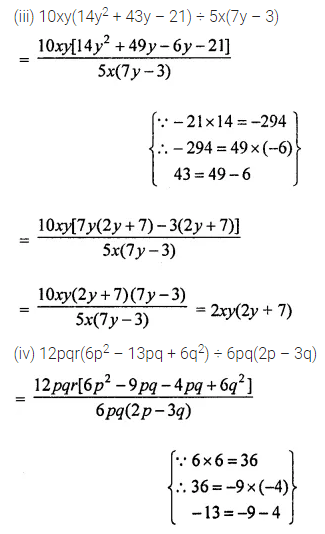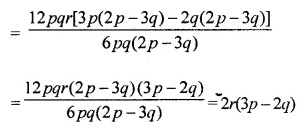# ML Aggarwal Class 8 Solutions for ICSE Maths Chapter 11 Factorisation Ex 11.5

## ML Aggarwal Class 8 Solutions for ICSE Maths Chapter 11 Factorisation Ex 11.5

Question 1.
Work out the following divisions:
(i) (35x + 28) ÷ (5x + 4)
(ii) 7p2q2(9r – 27) ÷ 63pq(r – 3)
Solution:Question 2.
Divide as directed:
(i) 6(2x + 7) (5x – 3) ÷ 3(5x – 3)
(ii) 33pq (p + 3) (2q – 5) ÷ 11p (2q – 5)
Solution:Question 3.
Factorise the expression and divide them as directed:
(i) (7x2 – 63x) ÷ 7(x – 3)
(ii) (3p2 + 17p + 10) ÷ (p + 5)
(iii) 10xy(14y2 + 43y – 21) ÷ 5x(7y – 3)
(iv) 12pqr(6p2 – 13pq + 6q2) ÷ 6pq(2p – 3q)
Solution: